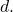# A note on the generators of the polynomial algebra of six variables and application

### Full PDFcarpathian_2023_39_2_529_539

Issue no:

Let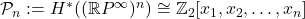be the graded polynomial algebra over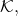where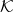denotes the prime field of two elements. We investigate the Peterson hit problem for the polynomial algebra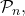viewed as a graded left module over the mod-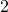Steenrod algebra,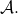For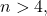this problem is still unsolved, even in the case of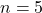with the help of computers.

In this paper, we study the hit problem for the case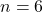in degree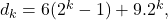with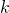an arbitrary non-negative integer. By consideringas a trivial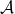-module, then the hit problem is equivalent to the problem of finding a basis of-graded vector space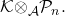The main goal of the current paper is to explicitly determine an admissible monomial basis of the-graded vector space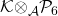in some degrees. At the same time, the behavior of the sixth Singer algebraic transfer in degree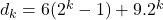is also discussed at the end of this article. Here, the Singer algebraic transfer is a homomorphism from the homology of the mod-Steenrod algebra,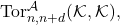to the subspace of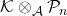consisting of all the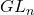-invariant classes of degree